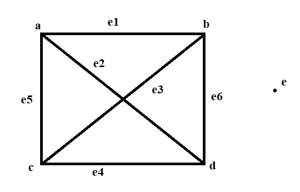# Maximum and minimum isolated vertices in a graph in C++

We are given with the number of edges Noe and number of vertices Nov. The goal is to find the minimum and maximum number of isolated vertices that are possible in such graphs which have no edges and No vertices count.

An isolated vertex is the one that has no edge connected to it.

• For minimum isolated vertices

We will make sure that every edge is isolated. ( No two edges have common vertices ) Each edge requires only 2 vertices. So ,

count of non isolated vertices = 2 * no. of edges

count of isolated vertices = total vertices - count of non isolated vertices.

If the no. of vertices is <= 2 * no. of edges, means all vertices have a edge connected. So no. of isolated vertices is 0.

• For maximum isolated vertices

For this we will try to make a polygon such that all edges are connected with minimum vertices.This is possible when we have a polygon such that each vertex pair also has diagonal between them.For given 5 vertices and 6 edges, the square is the polygon that has 6 edges with 2 diagonals so that only 4 vertices are occupied. 1 vertex becomes isolated and it is maximum.

No. of diagonals from one vertex to other in n sided polygon is n*(n-3)/2. Total edges=n*(n-1)/2

## Input

No. of vertices 5, edges 6

## Output

Minimum isolated vertices 0. Maximum isolated vertices 1.

## Explanation

As shown in above figure.

Input − no. of vertices 2, edges=1

Output − Minimum isolated vertices 0. Maximum isolated vertices 0.

Explanation − An edge is formed between at least two vertices.

## Approach used in the below program is as follows

• The integers noe and nov contains the no. of edges and vertices.

• Function findisolatedvertices(int v, int e) takes of edges and vertices as parameters and prints the minimum and maximum isolated vertices possible.

• If no. of vertices is <= 2*e, means no isolated vertex. Else non isolated vertices are 2*e (maximum), so minimum isolated will be v-2*e.

• For calculating maximum isolated vertices, start from i=1 to no. of vertices, if (i * (i - 1) / 2 >= e) break as only i vertices are sufficient for e edges.

• i stores the maximum isolated vertices possible.

## Example

Live Demo

#include <bits/stdc++.h>
using namespace std;
void findisolatedvertices(int v, int e){
//one edge has 2 vertices
if (v <= 2 * e) //means all veritces have a connected edge
cout << "Minimum no. of isolated vertices: " << 0 << endl;
else {
int niso=2*e; //maximum non isolated vertices
cout << "Minimum no. of isolated vertices: " << v - niso << endl;
}
// To find out maximum number of isolated
// vertices
// Loop to find out value of number of
// vertices that are connected
int i;
for (i = 1; i <= v; i++) {
if (i * (i - 1) / 2 >= e)
break;
}
cout <<endl<< "Maximum no. of isolated vertices: " <<v-i;
}
int main(){
// Number of vertices
int nov = 5;
// Number of edges
int noe = 2;
// Calling the function to maximum and
// minimum number of isolated vertices
findisolatedvertices(nov, noe);
return 0;
}

## Output

Minimum no. of isolated vertices: 1
Maximum no. of isolated vertices: 2